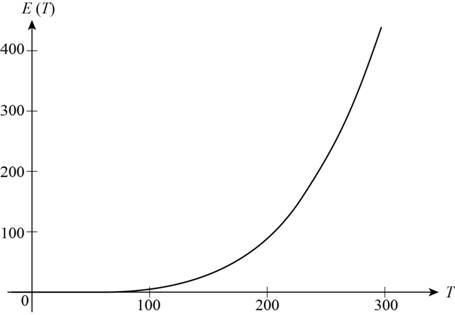# The graph of the function E ( T ) = ( 5.67 × 10 − 8 ) T 4 for temperature T between 100 K and 300 K.### Precalculus: Mathematics for Calcu...

6th Edition
Stewart + 5 others
Publisher: Cengage Learning
ISBN: 9780840068071### Precalculus: Mathematics for Calcu...

6th Edition
Stewart + 5 others
Publisher: Cengage Learning
ISBN: 9780840068071

#### Solutions

Chapter 2.3, Problem 51E

(a)

To determine

## To sketch: The graph of the function E(T)=(5.67×10−8)T4 for temperature T between 100 K and 300 K.

Expert Solution

### Explanation of Solution

The given function is E(T)=(5.67×108)T4 ,

Where,

• E is the energy radiated per unit of surface area measured in Watts.
•  T is the absolute temperature in Kelvin.

Use graphing calculator to draw the graph of the Function E with values of T between 100 K and 300 K as shown below in Figure 1.Figure (1)

From Figure (1), it is noticed that the value of E(T) increases as T increases.

(b)

To determine

Expert Solution

### Explanation of Solution

From Figure (1), it is clearly visible that the graph rises for all the values of T between 100 K and 300 K. It means that the function E is increasing on the interval (100,300) . Therefore, the energy radiated per unit of surface area increases as the temperature increases.

### Have a homework question?

Subscribe to bartleby learn! Ask subject matter experts 30 homework questions each month. Plus, you’ll have access to millions of step-by-step textbook answers!